﻿ Temperature Dependence of Viscosity and Surface Tension in Zn-Cd Liquid Alloy Using Optimization MethodPublications are Open
Access in this journal
Article Versions
Export Article
• Normal Style
• MLA Style
• APA Style
• Chicago Style
Research Article
Open Access Peer-reviewed

### Temperature Dependence of Viscosity and Surface Tension in Zn-Cd Liquid Alloy Using Optimization Method

H. K. Limbu , G.P. Adhikari
International Journal of Physics. 2020, 8(3), 81-89. DOI: 10.12691/ijp-8-3-1
Received June 16, 2020; Revised July 17, 2020; Accepted July 27, 2020

### Abstract

We have computed interchange energy by estimating the best fit experimental and theoretical values at 800K using Flory’s model. Taking these values at 800K, interchange energy at different temperatures are calculated using optimization method and with the help of which free energy of mixing, heat of mixing and entropy of mixing are obtained. The partial excess free energy is calculated with the help of values of free energy of mixing which is used to find surface tension. Butler’s equation and Layered structure approach have been used to calculate surface tension of Zn-Cd alloy. Viscosity is obtained from Singh and Sommer’s formulation, Moelwyn-Hughes equation, and Kaptay equation.

### 1. Introduction

In metallurgical science, the study of surface and transport properties of liquid alloy is important for the preparation of desired materials. Surface tension is required to understand the surface related phenomena such as wetting characteristics of solders, corrosion and kinetics of transformation. And, viscosity is important for many metallurgical process and heterogeneous chemical reactions. The different properties have been studied at 800K 1, here we have investigated surface tension and viscosity at different temperatures theoretically. We have used Butler’s equation 2 and Layered Structure approach 3, 4 to study the surface tension. The viscosity is studied with the help of Moelwyn - Hughes equation 5, Kaptay equation 4 and, Singh and Sommer’s Formulation 6.

Flory’s model 7, 8 has been used to explain the thermodynamic and structural behavior of the alloy at different temperatures as the size effect is noticeable. In the model, interaction energy parameter is considered as temperature dependent and is determined by fitting experimental free energy of mixing at different concentrations. We have calculated free energy of mixing (GM), activity (a), heat of mixing (HM) and the entropy of mixing (SM) at different temperature which are useful to determine surface tension and viscosity of the alloy. The formalism is presented in section 2, result and discussion is dealt in section 3, and conclusions are provided in section 4.

### 2. Formulation

The alloying behavior of liquid alloys can be studied with the help of either the electronic theory of mixing 9, 10 or the statistical mechanical theory of mixing. Metal physicists 11, 12, 13 have keen interest in explain the concentration dependent asymmetry in the properties of mixing of binary liquid alloys and hence to extract additional microscopic information.

Flory’s expression for the free energy of mixing of a binary mixture A-B consists of CA (≡ c) mole of A and CB {≡ c(1-c)} mole of B respectively, where CA and CB are the mole fractions of A (≡ Zn) and B (≡ Cd) in the binary liquid alloy A-B. The free energy of mixing of those alloys whose constituent atoms differ widely in size can be expressed as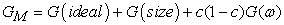(1)

Where,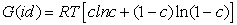(2)

G(size) and G(ω) are contributions due to the size effect and the interchange energy respectively which can be written from Flory’s model as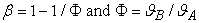(3)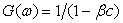(4)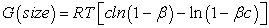(5)

Where,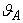and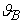are atomic volumes of the pure species A and B respectively 7 defined as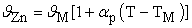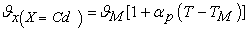(6)

Where,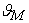= atomic volume at melting point

TM = melting temperature and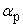= volume coefficient at constant temperature.

From Flory’s model the expression for free energy of mixing is given by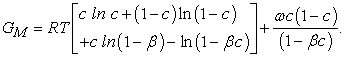(7)

The activity aA of the element A in the binary liquid alloy is given as,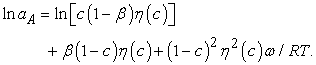(8)

The temperature derivative of GM gives an expression for integral entropy of mixing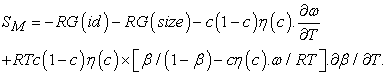(9)

Where,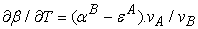(10)

and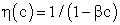(11)

Where,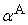and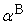are the coefficients of thermal expansion of pure species A and B respectively.

First term, second term, third term, and fourth tem in the right hand side of the equation (9) are due to ideal term, size factor (ф), temperature derivative terms of size factor, and interchange energy respectively. The necessity of taking ω as temperature dependent was noticed 9, 14.

Now, heat of mixing can be obtained from equation (1) and (9) from standard thermodynamic relation,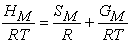(12)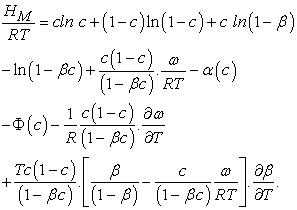(13)

Where,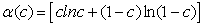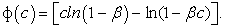2.1. Viscosity

The Moelwyn - Hughes equation:-

In order to examine the atomic transport behaviour, we have the Moelwyn-Hughes equation 5. The Moelwyn-Hughes equation for viscosity of liquid mixture is given as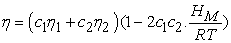(14)

Where,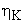(K=1, 2) is the viscosity of pure component K and for most liquid metals, it can be calculated from Arrhenius type equation 15 at temperature T as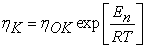(15)

Where,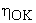is constant (in unit of viscosity) and En is the energy of activation of viscous flow for pure metal (in unit of energy per mole).

Singh and Sommer’s Formulation:-

Singh and Sommer 6 discussed the deviation in the viscosity of a binary liquid alloy from the ideal mixing quantitatively in terms of the energetic and the size factor. It is expressed as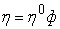(16)

Where,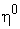is prefactor and ф is a function of composition.

Moelwyn - Hughes 5 assumed a linear behaviour of the prefactor,and approximated as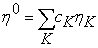(17)

Where,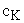andare the concentration of component and viscosity of pure component k respectively. Osman and Singh 16 suggested that when entropic effects are considered, the factor ф, as function of composition, can be obtained from the expression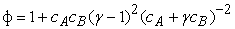(18)

Where,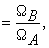B > ΩA, ΩK is atomic volume.

Kaptay equation:-

The Kaptay equation 17 for the viscosity of binary mixture has been derived taking account into the theoretical relationship between the activation energy of viscous flow. It is expressed as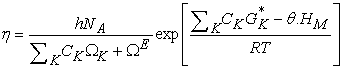(19)

Where, h = Plank’s constant, NA is Avogadro’s number, ΩK represents the molar volume, ΩE is the excess molar volume upon alloy formation, R is the ideal gas constant,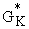is the Gibb’s energy of activation of the viscous flow in pure component K, HM is enthalpy of mixing of the alloy, CK (=A, B) represents concentration, and Ө is a constant whose value is taken to be 0.155±0.015 4.of component K is expressed as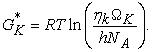(20)

The variation of viscosity,with temperature T from the Arrhenius type equation 16 is expressed as(21)

Where,is constant (in unit of viscosity) and En is the energy of activation of viscous flow for pure metal (in unit of energy per mole).

2.2 Surface Tension

Butler’s equation: -

Butler’s equation 2 is based on the assumption that the existence of surface monolayer at the surface of a liquid as a separate phase that is in thermal equilibrium with the bulk phase. It is expressed as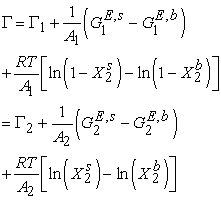(22)

Where, Γ1 and Γ2 are the surface tension of the pure component 1 and 2 respectively.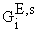and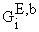(i = 1, 2) are partial excess free energies,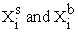are mole fraction of component i in the surface and bulk respectively. The molar surface area of the component i can be computed by using the relation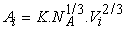(23)

Where, K (= 1.091) is geometrical factor for the liquid alloy 17. For binary mixture,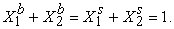Layered Structure approach:-

The layered structure approach 3, 4 connects the surface tension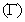of alloy to its bulk thermodynamic properties through the bulk activity coefficients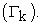It can be derived as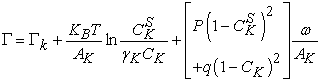(24)

Where, KB stands for Boltzmann constant;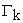is surface tension, CKS is the surface concentration of pure component K (K = A, B) at temperature T, p and q are surface coordination fractions, related as p + 2q = 1. In a simple cubic crystal z = 6, p = 2∕3 and q = 1∕6, in a bcc crystal z = 8, p = 3∕5 and q = 1∕5, and in a closely packed crystal, z = 12, p = 1∕2 and q = 1∕4 3, 4. And,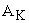is the mean area of the surface per atom which can be computed as AK=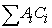( i= A,B), Where,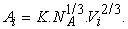2.3. Optimization of Free Energy of Mixing (GM), Activity (a), Heat of Mixing (HM), Surface Tensionand Viscosity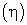The optimization method is a statistical thermodynamics or polynomial expressions. This method is useful to find theoretical values when the experimental values are unknown. At temperatures 700K, 950K and 1050K, the experimental values are unknown. So the method is suitable for our study.

The adjustable coefficients, used in the process, are estimated by least square method 18. The heat capacity can be expressed as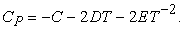(25)

From the thermodynamic relation, the enthalpy is given by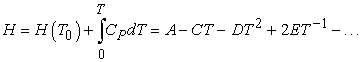(26)

Also, the entropy is given by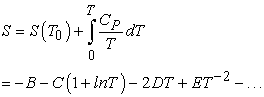(27)

By using equation (18) and (20) in the thermodynamic relation, G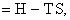we get the temperature (T) dependent free energy as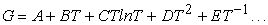(28)

The composition dependence of excess free energy of mixing is given by Redlich - Kister polynomial equation as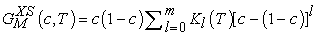(29)

With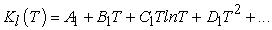(30)

The coefficients K1 depends upon the temperature same as that of G in equation (22). The least-square method can be used to obtain the parameters involved in equation (23). For this purpose, the required excess free energy of mixing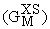of the liquid alloy at different temperatures can be determined by the relation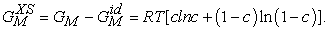(31)

The values for the free energy of mixing (GM) of liquid alloys at different temperatures can be calculated from equation (1) by knowing the values of ordering energy parameter (ω) at different temperatures from the relation 19, 20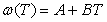(32)

Where, A and B are coefficient constants. The values of A and B are calculated with the help of values of ω/RT and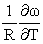at temperature 800K for the alloy using equation (32). The values ω/RT andat temperature 800K is computed using the best fit approximation of experimental values of the alloys from Hultgren et.al. 1973 21 using equations (7) and (9). By taking the values of coefficient constants A and B for the alloy, the values of interchange energy (ω) at different temperature T (i.e. T = 700K, 800K, 950K, and 1050K) are calculated.

### 3. Results and Discussion

The best fit parameters for Zn-Cd at 800K is found to be ω/RT = 1.058, and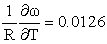using equation (7) and (9). By using the best fit value of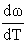and ω(T) at 800K for Zn-Cd in equation (32), the value of ω(T) at different temperatures are estimated and listed in Table 1.

The values of free energy of mixing (GM) of the liquid alloy at temperature T have been calculated by using the corresponding values of ω(T) in equation (7) over the entire range of concentration. And, the values of free energy of mixing (GM) are used to calculate the corresponding excess free energy of mixingof the alloy at temperatures of study by using equation (31).

Interaction energy (ω) is found to be positive which indicates segregating in nature. Using above interchange energy (ω), we have computed free energy of mixing (GM), activity (a), entropy of mixing (SM), heat of mixing (HM), surface tension (Γ) and viscosity (η) at temperatures 700K, 800K, 950K, 1050K using Flory’s model in which size factor plays important role.

• Figure 1. Graph for (GM/RT) versus the concentration of CZn of Zn-Cd liquid alloy at temperatures 700K, 800K, 950K and 1050K.
3.1. Free Energy of Mixing (GM)

The experimental values of free energy of mixing (GM) are taken from Hultgren et.al. 21 at 800K by which we have estimated the interchange energy (ω) at that temperatures by best fit method using equation (7) i.e. ω = 1.0850RT. The free energy of mixing (GM) at different temperatures are obtained using equation (7) with the help of optimized values of energy order parameters ω(T) presented on the Table 1. The plot of experimental values of free energy of mixing (GM) at 800K and theoretical values at different temperatures (T) of the alloy with respect to the concentration of Zn is shown in Figure 1.

3.2. Entropy of mixing(SM)

The experimental values of entropy of mixing(SM) at 800K for the alloy are taken for Hultgren et. al. 21. Using best fit method with the help of equation (9) the values ofestimated at temperature 800K i.e.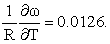The entropy of mixing of the alloy at different temperatures (T) are calculated using equation (9) conjugation with equations (10) and (11) with the help of optimized values of energy order parameters ω(T) presented on the Table 1 and constant values offrom equation (32) i.e.The plot of experimental values of entropy of mixing (SM) at 800K and at temperatures 700K, 800K, 950K, 1050K of the liquid alloy with respect to the concentration of Zn is shown in Figure 2.

• Figure 2. Graph for SM/R versus the concentration of CZn of Zn-Cd liquid alloy at temperatures 700K, 800K, 950K and 1050K.
3.3. Heat of Mixing (HM)

The heat of mixing (HM) for the alloy at temperatures 700K, 800K, 950K, 1050K are computed using equation (12) in conjugation with equations (7) and (9) with the help of corresponding optimized values of free energy of mixing (GM) and entropy of mixing (SM).

The plot of experimental values of heat of mixing (HM) at 800K and theoretical values at temperatures of study of the alloy with respect to the concentration of Zn is shown in Figure 3.

• Figure 3. Graph for HM/RT versus the concentration of CZn of Zn-Cd liquid alloy at temperatures 700K, 800K, 950K and 1050K.
3.4. Excess Free Energy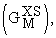Activity (a) and Partial Excess Free Energy (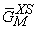)

The least-square method has been used to calculate the parameters involved in equation (29) for the alloy at different temperatures and then the optimized coefficients for the alloys are computed which are listed in the Table 2.

We have used the parameters i.e. Ko, K1, K2 and K3 to obtain partial excess free energy using equation (29). The partial excess free energy of mixing () of the components A (=Zn) and B (=Cd) are computed using equations 19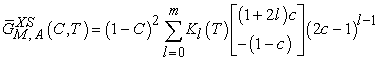(33)

and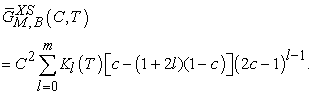(34)

The partial excess free energy of mixing of the components i.e. Zn and Cd involved in Zn-Cd, at temperature T have been calculated separately over the entire concentration range by equations (33) and (34) with the help of optimized coefficients i.e. Ko, K1, K2 and K3. The values of partial excess free energy of mixing of the components in the alloy at temperatures 700K, 800K, 950K, and 1050K are shown in Table 3, Table 4, Table 5 and Table 6.

Using these optimized partial excess free energy of mixing of both the components of the alloy we have calculated the corresponding excess free energy of the alloy at different temperatures over the entire range of concentration from the relation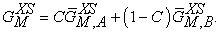(35)

The optimized values of excess free energy of mixing for the alloys at temperatures 700K, 800K, 950K and 1050K over the entire concentration range for Zn-Cd alloy is shown in Figure 4.

• Figure 4. Graph for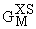/RT versus CZn of Zn-Cd liquid at temperatures 700K, 800K, 950K and 1050K

Now, the activity coefficients (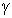i), (i =Zn or Cd) at different temperature over the entire range of concentration for components have been computed from the relation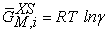(36)

With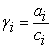(37)

Where, ai and ci be the activity and concentration of the components of liquid alloy at corresponding temperature. Using equations (36) and (37) we have computed activities of the components of alloys at different temperatures with the help of optimized values of partial excess free energy of the components that are presented on the Table 3, Table 4, Table 5 and Table 6. The plot of activity is presented on graph (5).

• Figure 5. Graph for at different temperatures versus CZn of Zn-Cd liquid alloy at 700K, 800K, 950K and 1050K
3.5. Surface Tension

The surface tension of the liquid alloys can be calculated using equation (22) conjugation with equation (23) using Butler’s model 2, 20. The ratio of partial excess Gibbs energy in the bulk and that in the surface can be expressed as

The value of parameter β has been taken as 0.83 as suggested by different researchers to compute surface tension of liquid alloys i.e. Guggenheim 11, Yeum et al. 22 and Tanaka et al. 17. The temperature coefficients for pure Zn and Cd components, surface tension at melting points of the components Zn and Cd are taken from Smithells Metal reference book 14. The surface tension of the pure component at temperature of investigation have been calculated using the relation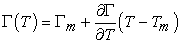Where,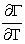(= -0.17mNm-1k-1 for Zn, and -0.26 mNm-1k-1 for Cd) is temperature coefficient of surface tension, Tm (= 692.5K and 594K for Cd) is melting temperature and T=700K, 800K, 950K and 1050K.

We have calculated surface concentration of Zinc corresponding to the bulk concentration of Zinc component in the alloys using Butler’s equation (22). The plot of the surface concentration of Zinc Vs bulk concentration of Zinc of Zn-Cd alloy at temperatures 700K, 800K, 950K and 1050K is shown in Figure 6.

• Figure 6. The surface concentration of Zn versus bulk concentration of Zn in Zn-Cd alloy at temperature 700K, 800K, 950K and 1050K using Butler’s equation

It is found from the analysis that the computed surface tension for Zn-Cd system at 800K is more than ideal value (= X1Γ1 + X2Γ2) at the concentrations from CZn=0.1 to 0.9 at 800K. The surface tension of Pure Zinc component and pure Cadmium component at melting point 14 is taken to calculate the surface tension at different temperatures. The surface tension of pure Zinc component is greater than the surface tension of pure Cadmium component at temperature T. We have calculated the surface tension with the concentration of Zinc component in the alloy using Butler’s equation (22) which indicates that as the concentration of Zinc component increases, surface tension increases at temperature T which is shown in Figure 7.

• Figure 7. The surface tension () versus concentration of Zn at temperatures 700K, 800K, 950K, 1050K using Butler’s equation

The surface tension of the alloy is calculated using Layered structure approach 3, 4 using equation (24) at temperature T. The plot of surface concentration of Zinc (CZnS) versus the bulk concentration of Zinc (CZn) of the alloy is shown in Figure 8 using Layered structure.

• Figure 8. Surface concentration of Zinc (CZnS) versus the bulk concentration of Zinc (CZn) of the alloy Zn-Cd alloy at temperatures 700K, 800K, 950K and 1050K using Layered Structure approach

We have computed the surface tension of the alloy using the values of surface concentrations which is shown in Figure 9 using Layered Structure approach at temperatures 700K, 800K, 950K and 1050K.

• Figure 9. The surface tension (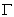) versus concentration of Zn of Zn-Cd alloy at temperatures 700K, 800K, 950K, 1050K using Layered Structure approach
3.6. Viscosity

The viscosity of the alloy at different temperatures(T) are computed using Moelwyn-Hughes equation 3, 23 with the help of heat of mixing (HM) which is shown in Figure 10. Viscosity of pure components i.e. Zn and Cd at temperature T (T =700K, 800K, 950K, 1050K) of the alloy are calculated using equation (15) with the help of the value of the constants E and ηok for the metals 15. And, heat of mixing (HM) at temperature T are obtained using equation (12) in conjugation with equations (7) and (9) with the help of optimized values of interchange energy parameters (ω) presented on Table 1.

• Figure 10. The viscosity of Zn-Cd liquid alloy versus concentration of Zinc at temperatures 700K, 800K, 950K, 1050K using Moelwyn-Hughes equation

The viscosity of the alloy at temperatures T are computed using Singh and Sommer’s formulation 6 with the help of equation (17) in conjugation with equation (18) taking the optimized values of heat of mixing (HM) at different temperatures (T). Viscosity of pure components i.e. Zn and Cd components in the alloy at temperature T of the alloy is calculated using equation (15) with the help of the value of the constants E and ηok for the metals 15. For simplicity, we have ignored the value of the excess molar volume (ΩE). The size factor plays important role to calculate viscosity which is computed using equation (6). The plot of the viscosity versus the concentration of Zinc component using Singh and Sommer’s formulation is shown in Figure 11.

• Figure 11. The viscosity of Zn-Cd liquid alloy versus concentration of Zinc at temperatures 700K, 800K, 950K, 1050K using Singh and Sommer’s Formulation

We have used equation (19) in conjugation with equations (20) and (21) to find the viscosity using Kaptay equation 4 with the help of optimized values of heat of mixing (HM) at different temperatures. Viscosities of pure components i.e. Zn and Cd components at temperature T of the alloy are calculated using equation (21) with the help of the value of the constants E and ηok for the metals 15. The plot of the viscosity versus the concentration of Zinc component using Kaptay equation is shown in Figure 12.

• Figure 12. The viscosity of Zn-Cd liquid alloy versus concentration of Zinc at temperatures 700K, 800K, 950K, 1050K using Kaptay equation

### 4. Conclusions

The interchange energy and size factor are temperature dependent in Zn-Cd alloy. Thermodynamic properties of the alloy are temperature dependent. The alloy is segregating and symmetric in nature at all temperatures of study. The surface tension and viscosity of the alloy are temperature dependent and decrease as the temperature of study increase at each concentration range. The optimization method is useful to obtain theoretical data at our temperatures of study where the experimental data is unavailable.

### References

  Mishra, K. K., Limbu, H. K., Yadav, B., Khan, A. K., Jha, I.S. and Adhikari, D., “Thermodynamic, structural, surface and transport properties of Zn-Cd liquid alloy at 800 K,” Bibechana NepJOL, vol. 14, pp. 54-65, 2017. In article View Article  Butler, J. A. V., “The Thermodynamics of the Surfaces of Solutions,” Proc. R. Soc. A Math. Phys. Eng. Sci., 135(827), 348-375, 1932. In article View Article  Guggenheim, E. A., “Mixture,” Oxford University press, Oxford, 1952. In article  Budai, I., Benko, M. Z. and Kaptay, G., “Comparison of different theoretical model to experimental data on viscosity of binary liquid alloys,” Matter. Sci. Forum, 537, 489-496, 2007. In article View Article  Moelwyn-Hughes, E. A., “Physical Chemistry” London: Longmans Green and co., Oxford, 1974. In article  Singh, R. N. and Sommer, F., “Thermodynamic investigation of viscosity and diffusion in binary liquid alloys,” Phys. Chem. Liq., 36, 17-28, 1998. In article View Article  Flory, P. J., “Thermodynamics of High Polymer Solutions,” J. Chem. Phys., vol. 10(1), 51, 1942. In article View Article  Limbu, H. K., Mishra, K. K., Adhikari, G. P. and Jha, I. S., “Theoretical investigation of Surface tension and Viscosity of Zinc based alloys at different temperatures using Optimization method,” International Journal of Theoretical and Mathematical Physics, Vol. 10(2), 33-41, 2020. In article  Harrison, W. A., “Pseudo potential in the theory of metals,” New York: Benjamin, 1966. In article  Bhatia, A. B., Hargrove, W. H. and March, N. H., “Concentration fluctuations in conformal solutions and partial structure factor in alloys,” J. Phys. C Solid State Phys. vol. 6(4), 621-630, Feb.1973. In article View Article  Koirala, I., “Chemical ordering of Au-Ag alloys in the molten state,” Journal of Institute of Science and Technology, 22(2), 191-201, 2018. In article View Article  Mishra, K. K., Limbu, H. K., Jha, N. K., Jha, I. S., Singh, B. P. and Adhikari, D., “Theoretical Investigation on Ordering Nature of Cd-Mg Alloys in Molten State,” J. Adv. Phys., 6(3), 449-455, 2017. In article View Article  Shrestha, G. K., Jha, I. S. and Singh, B. K., “Theoretical investigations on temperature dependence of thermodynamic properties and concentration fluctuations of In-Tl binary liquid alloys by optimization method,” Bibechana Nep JOL, 15, 11-23, 2018. In article View Article  Limbu, H. K., Mishra, K. K., Joshi, A. K., Magar, M. P., Jha, N. K., Jha, I. S. and Adhikari, D., “Theoretical investigation of thermodynamic, transport and structural properties of Au-Ag liquid alloy at different temperature.” International Journal of Scientific & Engineering Research, 9(5), 1181-1188, 2018. In article  Brandes, E. A. and Brook, G. B., “Smithells Metals Reference Book 7th edition,” Butterworth-Heinemann Reed Educational and Professional Publishing Ltd, Oxford, 1992. In article  Osman, S. M. and Singh, R. N., “Description of concentration fluctuations in liquid binary mixtures with non-additive potentials,” Phys. Rev. E., 52, 332-338, 1995. In article View Article  PubMed  Tanaka, T., Hack, K., Iida, T., and Hara, S., “Application of thermodynamic databases to the evaluation of surface tensions of molten alloys salt mixtures and oxide mixtures,” Zeitschrift Fuer Met., 87, 380-389, 1996. In article  Singh, R. N. and Sommer, F., “Segregation and immiscibility in liquid binary alloys,” Reports Prog. Phys., 60(1), 57-150, 1997. In article View Article  Singh, R. N. and Bhatia, A. B., “Flory’s formula for the entropy of mixing of NaCs alloy,” J. Phys. F: Met. Phys. 14, 2309-2314, 1984. In article View Article  Shrestha, G. K., Singh, B. K., Jha, I. S. and Koirala, I., “Theoretical study of thermodynamic properties of Cu-Pb liquid alloys at different temperature by optimization method,” Journal of Institute of Science and Technology, 22(1), 25-33, 2017. In article View Article  Hultgren, R., Desai, P. D., Hawkens, D. T., Gleisser, M. and Kelly, K. K., “Selected values of the Thermodynamic Properties of Binary Alloys,” American Society for Metals (ASM), Ohio, 430, 1973. In article  Yeum, K. S., Speiser, R., and Poirier, D. R., “Estimation of the surface tensions of binary liquid alloys.” Metallurgical Transaction B, 20, 693-703, 1989. In article View Article  Limbu, H. K., Mishra, K. K., Nirala, J., Jha, I. S., Singh, B. P. and Adhikari, D., “Thermodynamic, Microscopic and Surface Properties of Zn-In Liquid Alloy at 700 K,” IOSR Journal of applied Physics (IOSR-JAP), 9(1), 58-66, 2017. In article View ArticleThis work is licensed under a Creative Commons Attribution 4.0 International License. To view a copy of this license, visit http://creativecommons.org/licenses/by/4.0/